Anúncio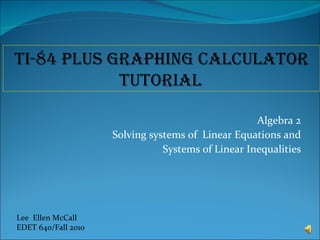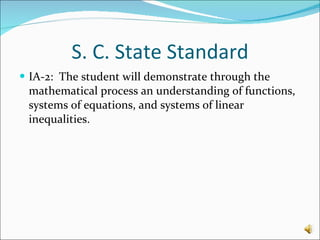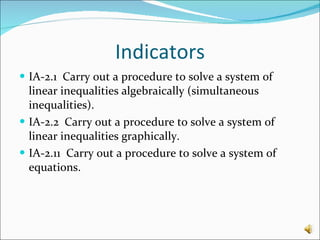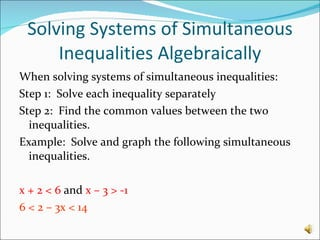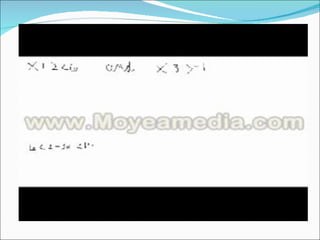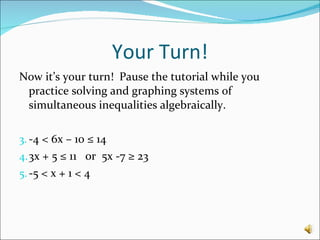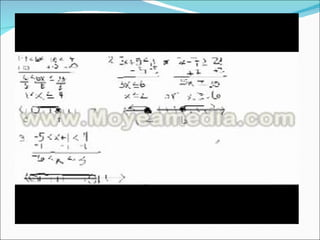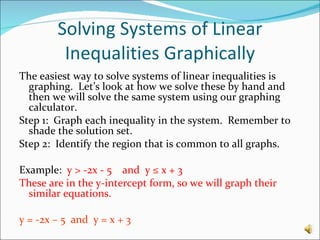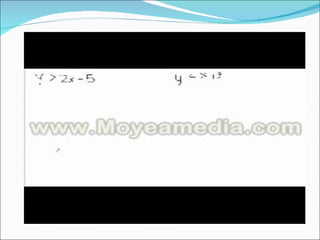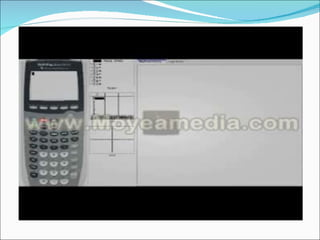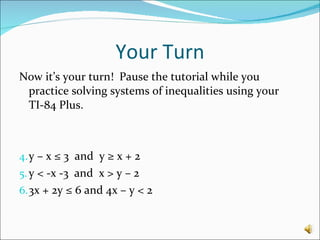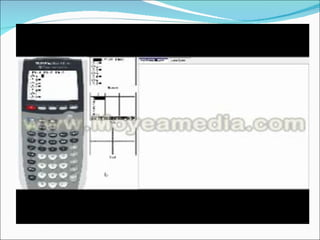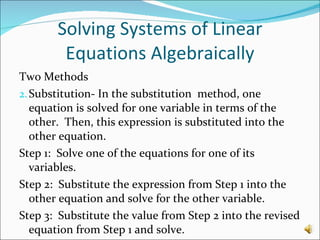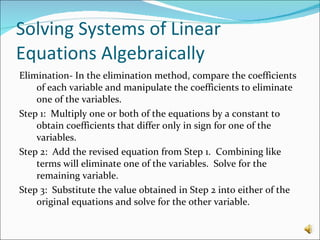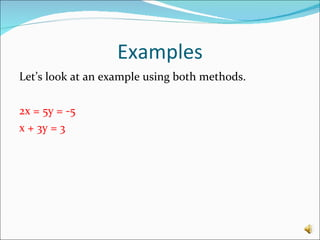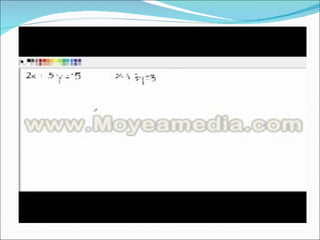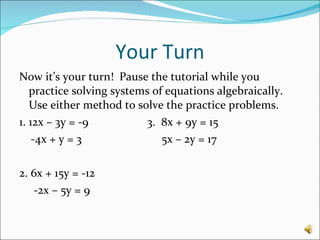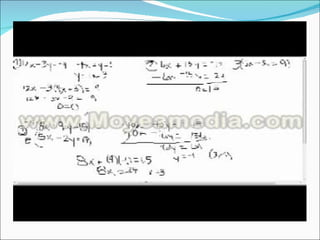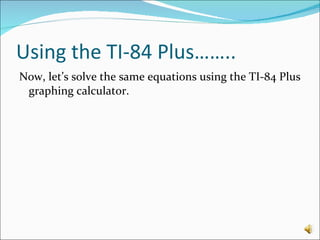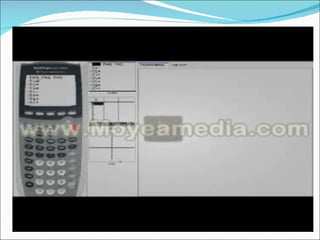1 de 21
Anúncio

### Mc call tutorial

1. Algebra 2 Solving systems of Linear Equations and Systems of Linear Inequalities Lee Ellen McCall EDET 640/Fall 2010 TI-84 Plus Graphing Calculator Tutorial
2.
3.
4.
5.
6.
7.
8.
9.

### Notas do Editor

1. Hello Everyone and welcome to this TI-84 plus graphing calculator tutorial. This tutorial is specifically for the algebra 2 standards that address solving systems of linear equations and linear inequalities. If your graphing calculator is not a TI-84 plus but is a Ti graphing calculator such as 83 or 83 plus, most of the keystrokes that I demonstrate will be exactly the same for you and you can easily follow along. You should all have a handout, a calculator, a pencil and possibly some scratch paper. Be ready to pause the tutorial for time to practice each skill when indicated. Let’s begin!
2. The South Carolina state standard this lesson covers is standard one a-2:
3. Within this standard, three of the listed indicators will be addressed with this lesson.
4. What is a solution? For a simultaneous inequality, the solution will be a range of data that satisfies both inequalities. We will address the first indicator: IA-2.1 Carry out a procedure to solve a system of linear inequalities algebraically (simultaneous inequalities).
5. Now, we will address the second objective: IA-2.2 Carry out a procedure to solve a system of linear inequalities graphically. What is a system of inequalities or equations? A system of linear equations or linear inequalities consists of at least two equations in two variables of the form Ax + By = C. What is the solution of a system of linear inequalities? The solution, if there is one, will be an ordered pair that is a solution of each inequality in the system. The graph of a system of inequalities is a graph of all solutions of the system, or the shaded area that is common to both inequalities. The easiest way….. Remember, these are linear, so we graph them the same way we would a line, with the inequality telling us whether the line is dashed or solid. If the inequality has the equal sign in it, the line is solid. If it is just less than or greater than, the line is dashed. Both inequalities include equal, so we know our line will be solid for both inequalities.
6. Now, it’s time to address the third objective: IA-2.11 Carry out a procedure to solve a system of equations. What is the solution of a system of linear equations? The solution is the point where the lines cross. This coordinate point is true for both equations. There are three possibilities when graphing systems of linear equations. 1. The lines cross at one point. We call this consistent and independent. If the lines are parallel (or have the same slope) they will never cross, so there is no solution and we call this inconsistent. 3. If the equations represent the same line, then one graph will fall on top of the other and we say there are infinitely many solutions so the lines are consistent and dependent. Remember: we can only solve systems that have the same variables. We are going to practice with systems in two variables.
7. As you can see, both of these equations have the variables x and y.
Anúncio